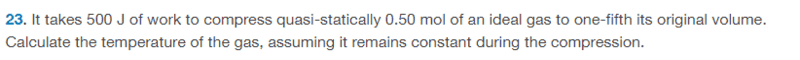# Work done to compress gas

• ChiralSuperfields

#### ChiralSuperfields

Homework Statement
Relevant Equations
PV = nRT
For this problem,dose anybody please give me guidance how they got 74 K as the answer? Note that chat GPT dose not give the correct answer (it gives the temperature of the gas is 1500 K).

Many Thanks!

This is a homework problem. You know the rules. Please show some work. I would also suggest that you look at the chat GPT answer and see whether there is anything you can use.

•ChiralSuperfields
This is a homework problem. You know the rules. Please show some work. I would also suggest that you look at the chat GPT answer and see whether there is anything you can use.

Since this is an ideal gas, I though I could you the ideal gas law. So as far as I got was setting the temperatures equal ##\frac{P_iV_i}{nR} = \frac{P_fV_i}{5nR}## which gave ##5P_i = P_f##.

Many thanks!

If you use calculus in your course, then your professor or textbook has probably derived a formula for the work associated with a quasi-static, isothermal expansion/compression of an ideal gas.

•ChiralSuperfields
If you use calculus in your course, then your professor or textbook has probably derived a formula for the work associated with a quasi-static, isothermal expansion/compression of an ideal gas.

No sorry, this course is algebras based. We did not do the calculus parts and alot of the thermo so stuff it not really making sense very much.

Many thanks!

If you use calculus in your course, then your professor or textbook has probably derived a formula for the work associated with a quasi-static, isothermal expansion/compression of an ideal gas.
Its an intro physics course so we cover dimensional analysis then thermo, and eventually mechanics and waves.

Nevermind, I think I really overthought this simple problem. Sorry.

Its an intro physics course so we cover dimensional analysis then thermo, and eventually mechanics and waves.
Ok. I wonder if the formula for isothermal work was given to you without a derivation. I don't see how to work the problem without this formula.

•Chestermiller and ChiralSuperfields
Ok. I wonder if the formula for isothermal work was given to you without a derivation. I don't see how to work the problem without this formula.
Thank you for your reply @TSny! Yeah, the only way I now realize that this problem to be solved is if we assume pressure it not constant, which then I have to use the work integral in terms of differential volume which was not shown in class. We were only shown ##W = P(V_2 - V_1)## which I now know assumes the special case where the pressure is constant.

•TSny